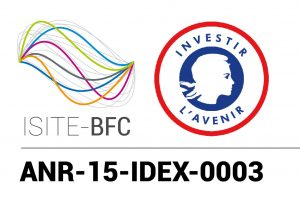#Innovation through Physics and Engineering

# MATH4PHYS

##Master in Mathematics for Physics (Math4Phys)

The recent progress in several fields of theoretical physics (such as high energy physics, astrophysics, quantum and nonlinear optics or condensed matter physics) required numerous very sophisticated mathematical tools. In these frontline research fields, it became clear that a new understanding of physical systems going from cold atom gases to black holes is impossible without a new insight into underlying mathematical structures. This kind of problems requires a new interdisciplinary approach and specialists with double competence: in Physics and in different fields of modern Mathematics.

The main aim of the Master Program In Mathematical Physics (Math4Phys) is to provide advanced lectures on the mathematical methods of modern theoretical physics in the framework of a mathematical curriculum. Such an offer exists in France only in Dijon as the Mathematical Physics group of the IMB (Burgundy Mathematical Institute) provides a unique environment for a program requiring a double competence in Mathematics and Physics. The Mathematical Physics group of the IMB laboratory in Dijon is a unique research team in France with a capacity to provide advanced lectures in mathematical problems of modern physics. It permits to create a scientific environment for a master program focused on the most important problems of modern Physics from the mathematical perspective.

We offer lecture courses for the students with background in mathematics or mathematical physics giving an introduction to the mathematical methods used for such branches of theoretical physics as quantum field theory, statistical mechanics, general relativity, gauge theories, string theory, etc. The coursework covers different fields of mathematics (algebra, geometry, analysis) and highlights their applications to the problems of modern theoretical physics. The students are integrated from the very beginning into the mathematical physics group of the IMB and have to prepare by the end of each year a master dissertation.

#### Curriculum

The first year (M1) of the program is designed to provide the necessary background courses (mostly in mathematics but also in physics) to comply with the coursework of the more advanced second year.

We will also provide several mini courses by the research visitors of IMB.

#### Main research areas

Courses of Math4Phys are covering different fields of mathematics (algebra, geometry, analysis) and highlighting their applications to the problems of modern theoretical physics, giving an introduction to the mathematical methods used for branches of theoretical physics such as:

• Quantum field theory
• Statistical mechanics
• General relativity
• Gauge theories
• String theory, etc.

#### Career paths

The main aim of the master program is to provide sufficient training to start a PhD thesis for a futur research career .

#### Entry requirements

The students applying for the M1 have to complete their undergraduate studies with major in Mathematics or Physics.

The students can apply directly for the second year (M2) if they have completed at least one year of graduate courses in Mathematics or Mathematical Physics.

Maximum enrolment 20 in M1 and 15 in M2.

To follow the program the students should have a sufficient proficiency in English (we don’t require TOEFL or an equivalent certificate but we can suggest an online interview to candidates).

#### Grants

Several fellowship grants (800 € per month, during up to 10 months) will be awarded each year to high quality students.

Contact: Nikolai KITANINE